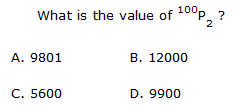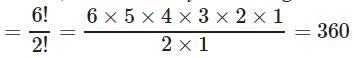Courses

# Test: Permutation And Combination- 1

## 10 Questions MCQ Test IBPS PO Mains - Study Material, Online Tests, Previous Year | Test: Permutation And Combination- 1

Description
This mock test of Test: Permutation And Combination- 1 for Quant helps you for every Quant entrance exam. This contains 10 Multiple Choice Questions for Quant Test: Permutation And Combination- 1 (mcq) to study with solutions a complete question bank. The solved questions answers in this Test: Permutation And Combination- 1 quiz give you a good mix of easy questions and tough questions. Quant students definitely take this Test: Permutation And Combination- 1 exercise for a better result in the exam. You can find other Test: Permutation And Combination- 1 extra questions, long questions & short questions for Quant on EduRev as well by searching above.
QUESTION: 1

### A bag contains 2 white balls, 3 black balls and 4 red balls. In how many ways can 3 balls be drawn from the bag, if at least one black ball is to be included in the draw?

Solution:

The bag contains 2 White, 3 Black and 4 Red balls.

So, total 9 balls are there in the bag; among them 3 are Black and 6 are non-Black balls.

Three balls can randomly be drawn in (9C3) = 84 ways.

1 Black and 2 non-Black balls can be drawn in (3C1)*(6C2) = 45 ways.

1 non-Black and 2 Black balls can be drawn in (6C1)*(3C2) = 18 ways.

3 Black balls can be drawn in (3C3) = 1 way.

So, three balls drawn in (45 + 18 + 1) = 64 ways will have at least one Black ball among the drawn ones.

QUESTION: 2

### A coin is tossed 3 times. Find out the number of possible outcomes.

Solution:

For any multiple independent event, there are nm

total possible outcomes, where n is the number of outcomes per event, and m is the number of such events.

So for a coin, discounting the unlikely event of landing on its side, there are two possible outcomes per event, heads or tails. And it is stated that there are 3 such events. So nm=23=8

.

QUESTION: 3

### .Solution:

We know , nPr = n ! /( n - r) !

So 100P= 100 ! / ( 100 - 2 ) !

= 100 ! / 98 !

= 100 x 99 x 98 ! / 98 !

= 9900

So Option D is correct answer.

QUESTION: 4

In how many ways can the letters of the word 'LEADER' be arranged?

Solution:

The word 'LEADER' has 6 letters.
But in these 6 letters, 'E' occurs 2 times and rest of the letters are different.
Hence,number of ways to arrange these lettersQUESTION: 5

How many 3 digit numbers can be formed from the digits 2, 3, 5, 6, 7 and 9 which are divisible by 5 and none of the digits is repeated?

Solution:

Since each desired number is divisible by 5, so we must have 5 at the unit place. So, there is 1 way of doing it.
The tens place can now be filled by any of the remaining 5 digits (2, 3, 6, 7, 9). So, there are 5 ways of filling the tens place.
The hundreds place can now be filled by any of the remaining 4 digits. So, there are 4 ways of filling it.
∴ Required number of numbers = (1 x 5 x 4) = 20.

QUESTION: 6

How many words with or without meaning, can be formed by using all the letters of the word, 'DELHI' using each letter exactly once?

Solution:

Explanation :

The word 'DELHI' has 5 letters and all these letters are different.

Total words (with or without meaning) formed by using all these
5 letters using each letter exactly once
= Number of arrangements of 5 letters taken all at a time
= 5P5 = 5! = 5 x 4 x 3 x 2 x 1 = 120

QUESTION: 7

In how many different ways can the letters of the word 'JUDGE' be arranged such that the vowels always come together?

Solution:

No. of vowels in the word JUDGE = 2 i.e., U and E.

In such cases we treat the group of two vowels as one entity or one letter because they are supposed to always come together. Thus, the problem reduces to arranging 4 letters i.e. J, D. G and [UE] in 4 vacant places.

No. of ways 4 letters can be arranged in 4 places = 4×3×2×1 = 24

But the 2 vowels can be arranged among themselves in 2 different ways by interchanging their position. Hence, each of the above 24 arrangements can be written in 2 ways.

Therefore, Required No. of total arrangements = 24×2 = 48

QUESTION: 8

How many arrangements can be made out of the letters of the word 'ENGINEERING' ?

Solution:

The number of arrangements of the word ENGINEERING is 277200.

Solution:

ENGINEERING word has 3 times of 3, three times of N, 2 times of G and 2 times of I. Then, the total letter is 11.

So, the number of arrangements of the word ENGINEERING = 11!/[3! * 3! * 2! * 2!]

= 39916800/[6 * 6 * 2 * 2]

= 277200

Hence, the number of arrangements of the word ENGINEERING is 277200.

QUESTION: 9

In how many different ways can the letters of the word 'DETAIL' be arranged such that the vowels must occupy only the odd positions?

Solution:

There are 6 letters in the given word, out of which there are 3 vowels and 3 consonants.

Let us mark these positions as under:

(1) (2) (3) (4) (5) (6)

Now, 3 vowels can be placed at any of the three places out 4, marked 1, 3, 5.

Number of ways of arranging the vowels = 3P3

= 3! = 6.

Also, the 3 consonants can be arranged at the remaining 3 positions.

Number of ways of these arrangements = 3P3

= 3! = 6.

Total number of ways = (6 x 6) = 36.

QUESTION: 10

How many words can be formed by using all letters of the word 'BIHAR'?

Solution:

Explanation :

The word 'BIHAR' has 5 letters and all these 5 letters are different.

Total words formed by using all these 5 letters = 5P5 = 5!
= 5 x 4 x 3 x 2 x 1 = 120